2023年3月4日

# # 美团 - 如何实现一个有序链表能够进行“二分“查找？

## # 1. 怎么理解跳表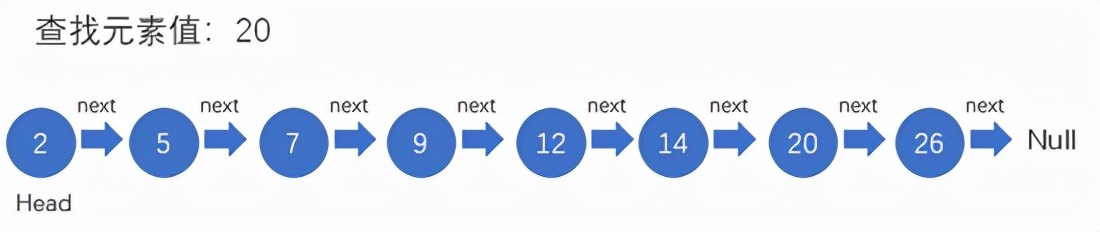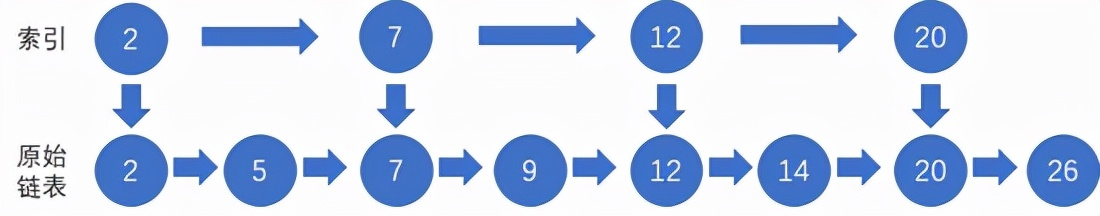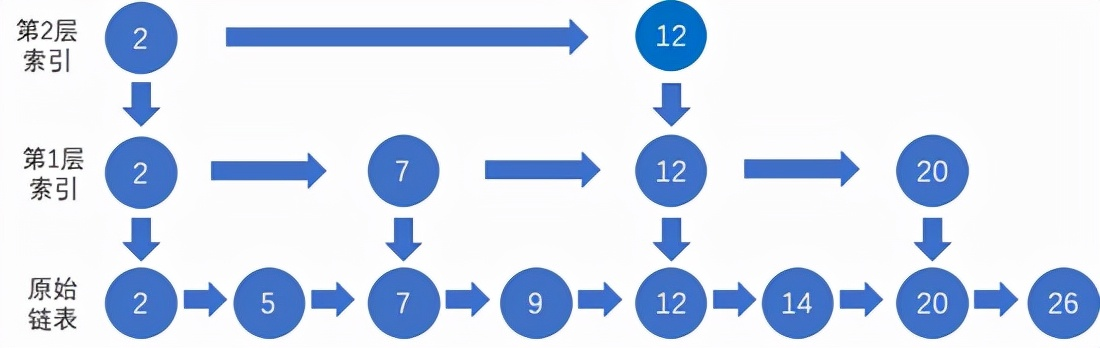## # 2. 跳表复杂度分析

### # 2.1. 时间复杂度

m的值怎么计算呢？在上面的例子中，每一层最多只需要遍历三个元素，因此m=3，根据 时间复杂度的计算规则 ，高阶的常数项也可以省略，因此跳表中查询任意数据的时间复杂度就是 O(logn)

### # 2.2. 空间复杂度

tips：这是一个典型的空间换时间操作。原始链表中存储的有可能是很大的对象，而索引结点只需要存储关键值和几个指针，并不需要存储对象，所以当对象比索引结点大很多时，索引占用的额外空间就可以忽略了。

## # 3. 高效的插入和删除

### # 3.1. 插入操作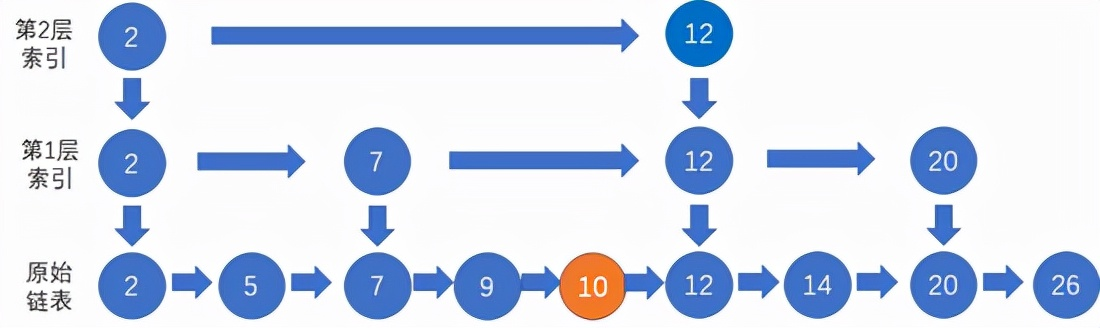### # 3.2. 删除操作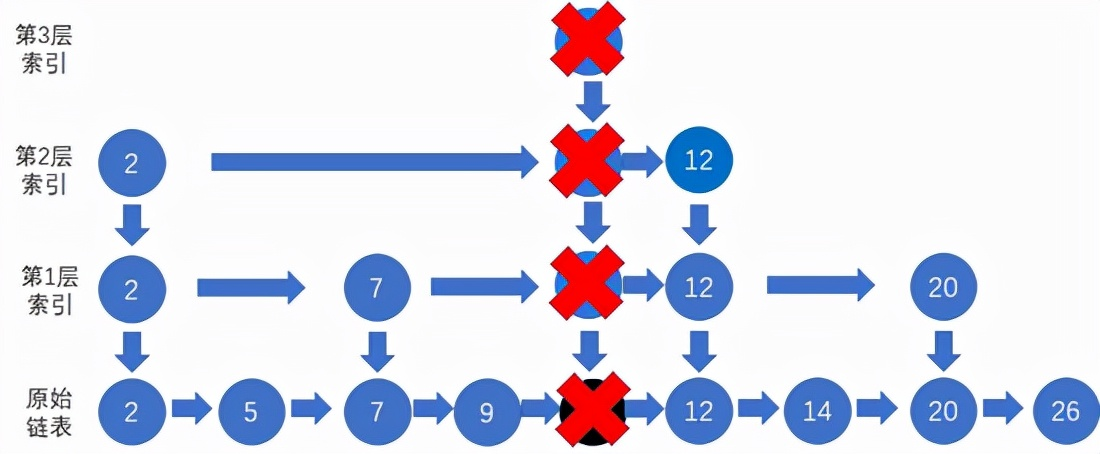## # 4. 动态更新索引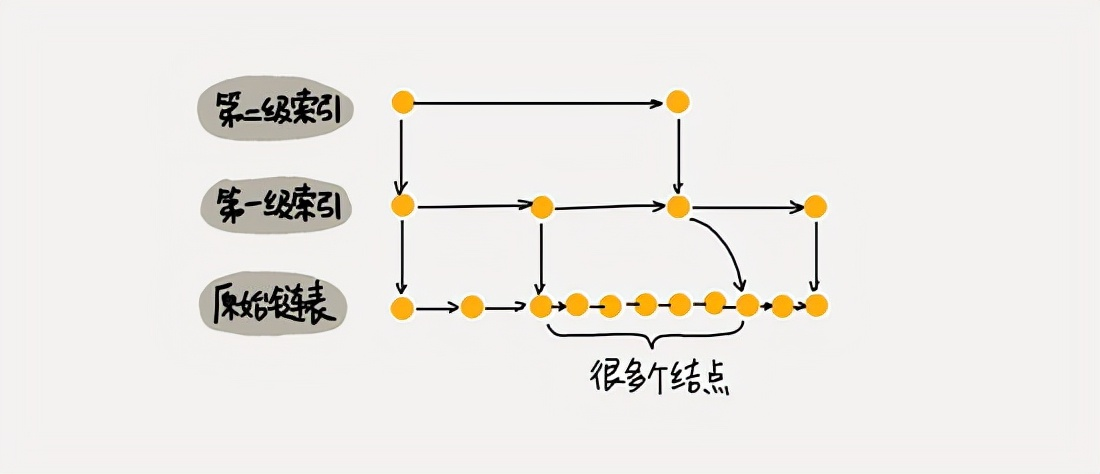## # 5. Java实现跳表

``````package skiplist;
/**
* 跳表的一种实现方法。
* 跳表中存储的是正整数，并且存储的是不重复的。
*
* Author：ZHENG
*/
public class SkipList {
private static final float SKIPLIST_P = 0.5f;
private static final int MAX_LEVEL = 16;

private int levelCount = 1;

private Node head = new Node();  // 带头链表

public Node find(int value) {
for (int i = levelCount - 1; i >= 0; --i) {
while (p.forwards[i] != null && p.forwards[i].data < value) {
p = p.forwards[i];
}
}

if (p.forwards != null && p.forwards.data == value) {
return p.forwards;
} else {
return null;
}
}

public void insert(int value) {
int level = randomLevel();
Node newNode = new Node();
newNode.data = value;
newNode.maxLevel = level;
Node update[] = new Node[level];
for (int i = 0; i < level; ++i) {
}

// record every level largest value which smaller than insert value in update[]
for (int i = level - 1; i >= 0; --i) {
while (p.forwards[i] != null && p.forwards[i].data < value) {
p = p.forwards[i];
}
update[i] = p;// use update save node in search path
}

// in search path node next node become new node forwords(next)
for (int i = 0; i < level; ++i) {
newNode.forwards[i] = update[i].forwards[i];
update[i].forwards[i] = newNode;
}

// update node hight
if (levelCount < level) levelCount = level;
}

public void delete(int value) {
Node[] update = new Node[levelCount];
for (int i = levelCount - 1; i >= 0; --i) {
while (p.forwards[i] != null && p.forwards[i].data < value) {
p = p.forwards[i];
}
update[i] = p;
}

if (p.forwards != null && p.forwards.data == value) {
for (int i = levelCount - 1; i >= 0; --i) {
if (update[i].forwards[i] != null && update[i].forwards[i].data == value) {
update[i].forwards[i] = update[i].forwards[i].forwards[i];
}
}
}

levelCount--;
}
}

// 理论来讲，一级索引中元素个数应该占原始数据的 50%，二级索引中元素个数占 25%，三级索引12.5% ，一直到最顶层。
// 因为这里每一层的晋升概率是 50%。对于每一个新插入的节点，都需要调用 randomLevel 生成一个合理的层数。
// 该 randomLevel 方法会随机生成 1~MAX_LEVEL 之间的数，且 ：
//        50%的概率返回 1
//        25%的概率返回 2
//      12.5%的概率返回 3 ...
private int randomLevel() {
int level = 1;

while (Math.random() < SKIPLIST_P && level < MAX_LEVEL)
level += 1;
return level;
}

public void printAll() {
while (p.forwards != null) {
System.out.print(p.forwards + " ");
p = p.forwards;
}
System.out.println();
}

public class Node {
private int data = -1;
private Node forwards[] = new Node[MAX_LEVEL];
private int maxLevel = 0;

@Override
public String toString() {
StringBuilder builder = new StringBuilder();
builder.append("{ data: ");
builder.append(data);
builder.append("; levels: ");
builder.append(maxLevel);
builder.append(" }");

return builder.toString();
}
}
}
``````

## # 6. 个人总结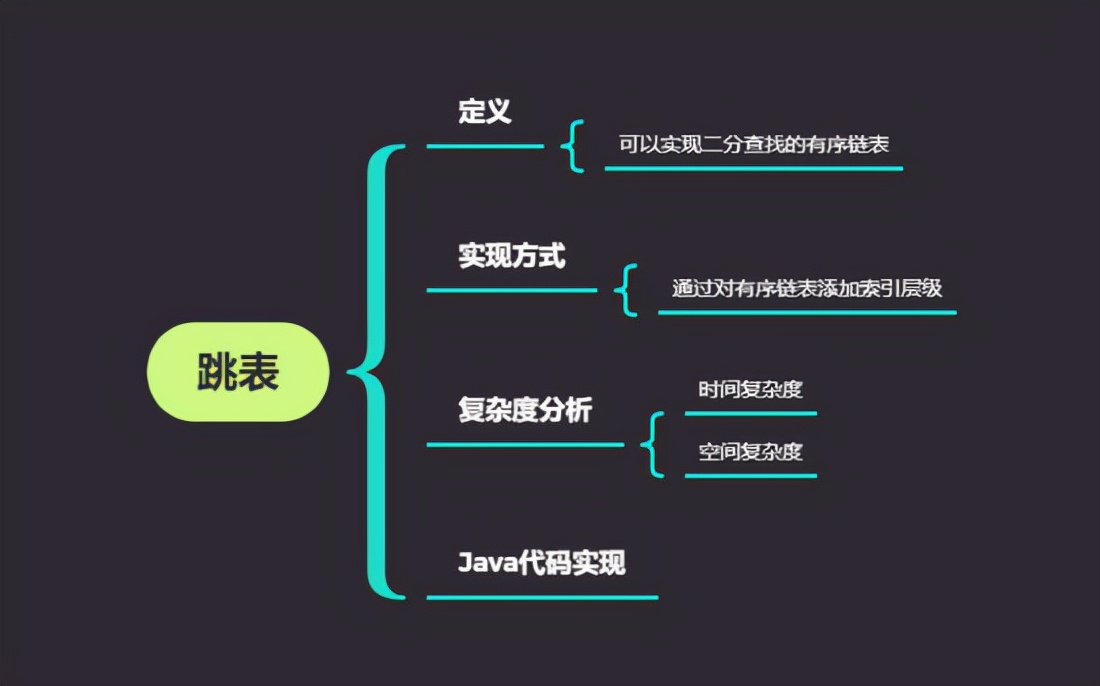## # 引用资料

• https://blog.csdn.net/m0_69305074/article/details/124069607